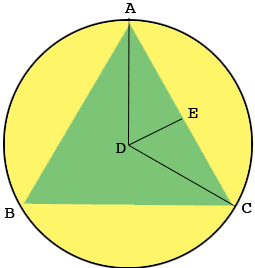SEARCH HOMEMath Central Quandaries & QueriesQuestion from Olatundun: An Equilateral Triangle Of Side 20cm Is Inscribed In A Circle. Calculate The Distance Of A Side Of The Triangle From the Centre Of The Circle.Hi,In my diagram, $D$ is the centre of the circle, $ABC$ is the inscribed equilateral triangle and $E$ is the midpoint of the line $CA$ so the length of $EA$ is 10 cm.

What is the measure of the angle $CAB?$

What is the measure of the angle $CAD?$

What is the measure of the angle $DEA?$

Penny* Registered trade mark of Imperial Oil Limited. Used under license.Math Central is supported by the University of Regina and the Imperial Oil Foundation.